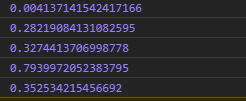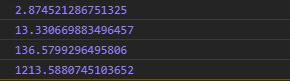# D3.js randomExponential() Function

The randomExponential() function is used to return a function that generates the random number based on the exponential distribution.

Syntax:

`d3.randomExponential(lambda);`

Parameters: It takes only one parameter that is given above and described below.

• lambda: It is the rate of the exponential distribution function which is equal to the time between events in a Poisson process.

Returns: It returns a function.

Note: The value of the output may be different each time the function is run.

Below given are a few examples of the above function.

Example 1: When lambda is greater than one.

 ` ` `<``html` `lang``=``"en"``> ` `<``head``> ` `  ``<``meta` `charset``=``"UTF-8"``> ` `  ``<``meta` `name``=``"viewport"`  `        ``content="``width``=``device``-width, ` `                 ``initial-scale``=``1``.0"> ` `  ``<``title``>Document ` ` ` `<``style``> ` ` ` `<``body``> ` `  ``` `  ``<``script` `type` `= ``"text/javascript"` `          ``src` `= ``"https://d3js.org/d3.v4.min.js"``> ` `  `` ` `  ``<``script``> ` `    ``/*  The value of the output may be ` ` ``different each time the function is run.*/ ` `    ``console.log(d3.randomExponential(5)()) ` `    ``console.log(d3.randomExponential(5)()) ` `    ``console.log(d3.randomExponential(5)()) ` `    ``console.log(d3.randomExponential(5)()) ` `    ``console.log(d3.randomExponential(5)()) ` `  `` ` ` ` ``

Output:Example 2: When the value of lambda is less than one.

 ` ` `<``html` `lang``=``"en"``> ` `<``head``> ` `  ``<``meta` `charset``=``"UTF-8"``> ` `  ``<``meta` `name``=``"viewport"`  `        ``content="``width``=``device``-width,  ` `                 ``initial-scale``=``1``.0"> ` `  ``<``title``>Document ` ` ` `<``style``> ` ` ` `<``body``> ` `  ``` `  ``<``script` `type` `= ``"text/javascript"`  `          ``src` `= ``"https://d3js.org/d3.v4.min.js"``> ` `  `` ` `  ``<``script``> ` `    ``/*  The value of the output may be ` ` ``different each time the function is run.*/ ` `    ``console.log(d3.randomExponential(0.5)()) ` `/* Smaller the lamba greater the random value generated. ` `    ``console.log(d3.randomExponential(0.05)()) ` `    ``console.log(d3.randomExponential(0.005)()) ` `    ``console.log(d3.randomExponential(0.0005)()) ` `  `` ` ` ` ``

Output:My Personal Notes arrow_drop_upCheck out this Author's contributed articles.

If you like GeeksforGeeks and would like to contribute, you can also write an article using contribute.geeksforgeeks.org or mail your article to contribute@geeksforgeeks.org. See your article appearing on the GeeksforGeeks main page and help other Geeks.

Please Improve this article if you find anything incorrect by clicking on the "Improve Article" button below.

Article Tags :

Be the First to upvote.

Please write to us at contribute@geeksforgeeks.org to report any issue with the above content.